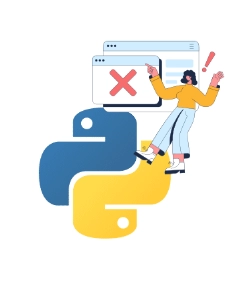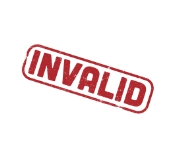New update is available. Click here to update.
Last Updated: Jun 30, 2023
Easy

# Invalid Syntax in Python: Common Reasons for SyntaxError

## Introduction

Let’s start discussing the invalid syntax in Python, which leads to Syntax errors. First of all, let us have a brief introduction to Python and the reasons behind this error.Python is written in a very layman language. A newbie coder could learn Python easily compared to any other language. So, what happens is if a person switches from another language, syntax changes which leads to confusion and results in the formation of error.

So, here we will be discussing all possible errors made. We will also discuss how to avoid them. Let’s start understanding what is invalid Syntax in Python or common reasons for syntax errors in Python.

You can also read about the Multilevel Inheritance in Python, Swapcase in Python

## Invalid Syntax in Python

While running our code, when the output doesn’t display on our screen, most certainly, it’s a syntax error.In other words, when the python interpreter fails to give the output on the screen, this arises the case of invalid syntax. The interpreter also shows the line number where the error has occurred. This helps in traceback and correcting errors.

Now we will be discussing common reasons for syntax errors in python.

### Missing of brackets

``print("Hello world."``

Here we can see that the output screen clearly shows that a bracket is missing at the end.

### Inconsistent indentation

``````def one():
return 1

def two():
return 2

print("Choose operation.")
print("1.Return 1")
print("2.Return 2")

while True:
choice = input("Enter 1 or 2: ")
if choice in ('1', '2'):
#incorrect indentation
if choice == '1':
print("1")

elif choice == '2':
print("2")

next = input("Print again? (yes/no): ")
if next == "no":
break

else:
print("Invalid Input")
``````

Indentation plays an important role in python code. Wrong indentation results in an error in Python. Here, the output screen indicates where indentation is wrongly used.

### Missing of Colon

``````#Missing of colon
def one()
return 1

def two():
return 2

print("Choose operation.")
print("1.Return 1")
print("2.Return 2")

while True:
choice = input("Enter 1 or 2: ")
if choice in ('1', '2'):
if choice == '1':
print("1")

elif choice == '2':
print("2")

next = input("Print again? (yes/no): ")
if next == "no":
break

else:
print("Invalid Input")

``````

After any function or compound statement such as if, else, while, for, the colon is required after the condition.

Also see,  Convert String to List Python

### Using keywords as a variable name

``````def one():
return 1

def two():
return 2

print("Choose operation.")
print("1.Return 1")
print("2.Return 2")

while True:
#keyword used as a variable name
def = input("Enter 1 or 2: ")
if def in ('1', '2'):
if def == '1':
print("1")

elif def == '2':
print("2")

next = input("Print again? (yes/no): ")
if next == "no":
break

else:
print("Invalid Input")``````

def is a keyword in Python. So, using it results in invalid syntax.

### Not Understanding the difference between “=” and “==”

``````def one():
return 1

def two():
return 2

print("Choose operation.")
print("1.Return 1")
print("2.Return 2")

while True:
def == input("Enter 1 or 2: ")
if def in ('1', '2'):
if def == '1':
print("1")

elif def == '2':
print("2")

next = input("Print again? (yes/no): ")
if next == "no":
break

else:
print("Invalid Input")
``````

“=” is used for assigning something, and “==” is used for checking the equality condition.

### Non-Matching Quotation marks

``````print("Hello World')
``````

If the string contained in quotation marks starts with double quotes, it should end with double quotes.

In Python code, indentation plays the most important part. Make sure that your code is well indented. You can try it on online python compiler.

It's time to discuss some FAQs related to the Invalid Syntax in Python.

### What do you mean by Syntax in Python?

Syntax generally refers to a set of rules predefined to be followed to come up with the correct output.

### How can one fix the invalid syntax in Python?

Read the error message properly. Tracebacks are good enough to find the reason for mistakes in your code.

### What are the different types of programming errors?

Basically, there are three different types of programming errors: Syntax error, Runtime error and logical errors.

### Why is Python used widely?

Python is a very simple programming language widely used in data analysis, mainly in Machine learning and artificial intelligence.

## Conclusion

We have discussed the Invalid Syntax in Python, including its example and the error that would be displayed on the output screen.

After reading about the Invalid Syntax in Python, are you not feeling excited to read/explore more articles on Data Structures and Algorithms? Don't worry; Coding Ninjas has you covered. See JavaBasics of JavaJava AWTJava AWT Basics, and Spring Boot to learn. Refer to our Guided Path on Coding Ninjas Studio to upskill yourself in Data Structures and AlgorithmsCompetitive ProgrammingJavaScriptSystem Design, and many more!

Do upvote our blogs if you find them helpful and engaging!

Happy Learning!